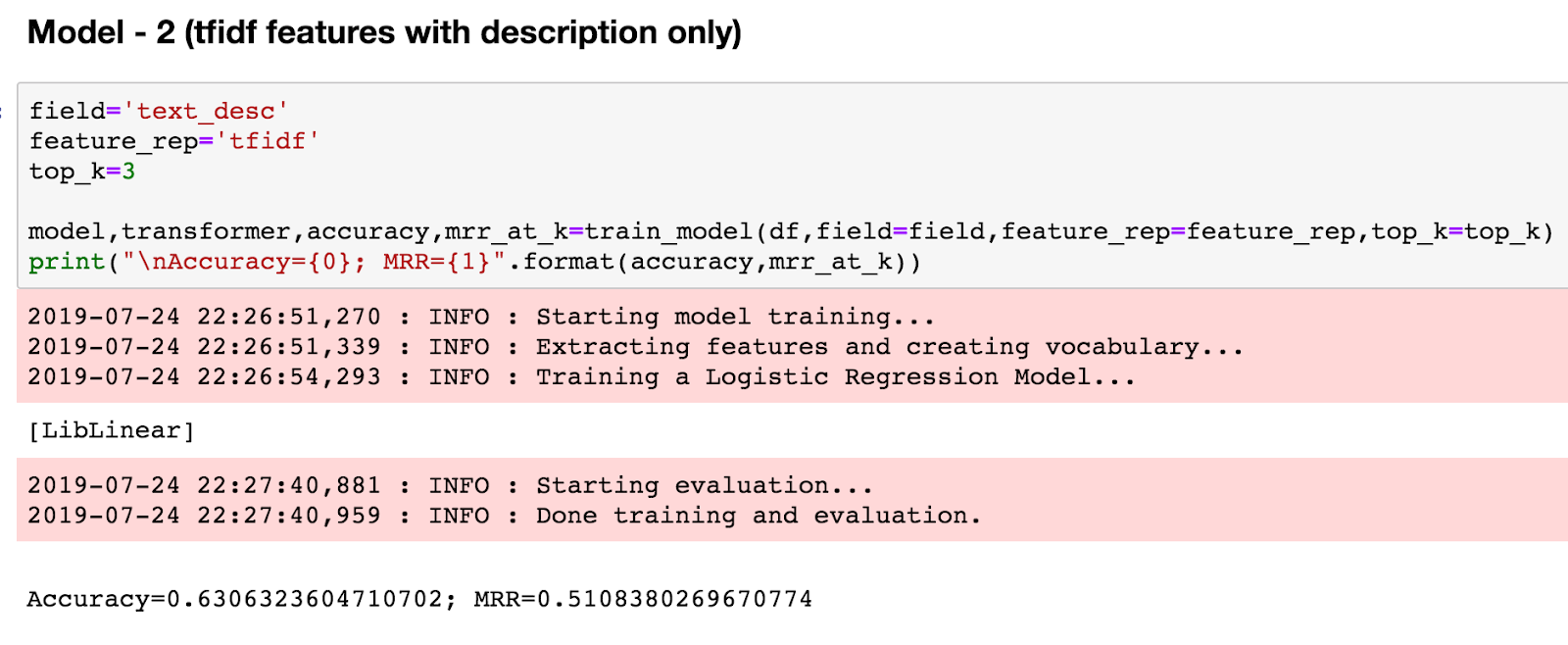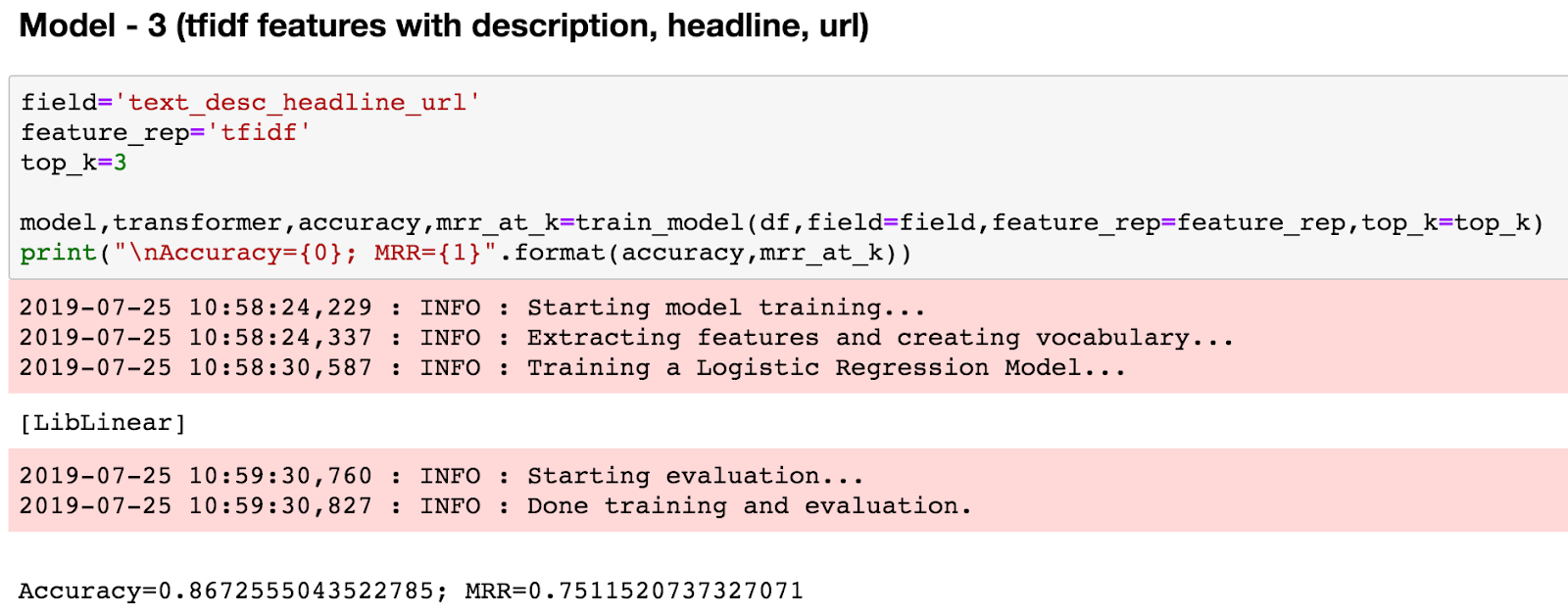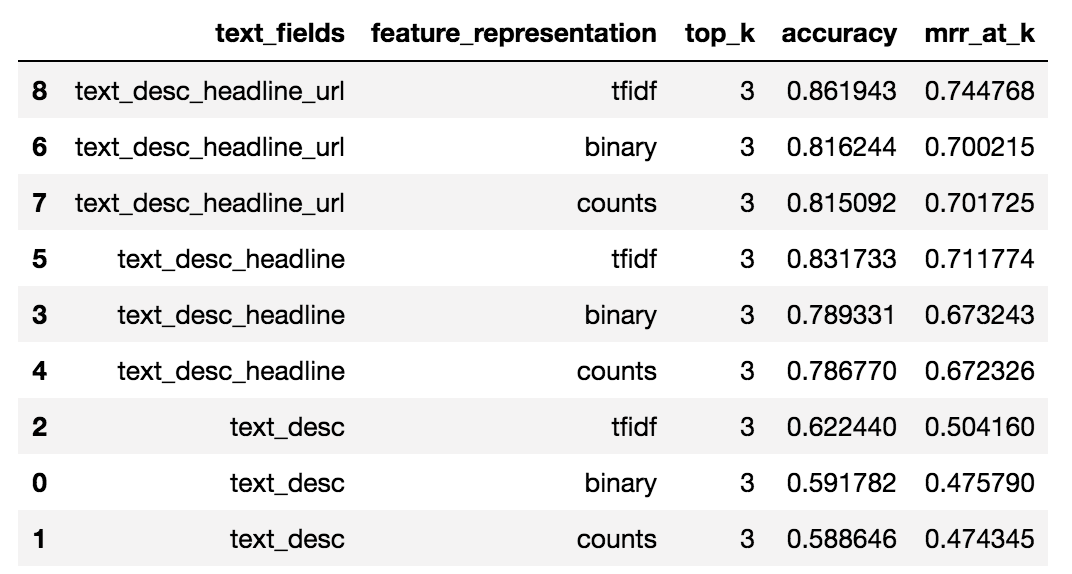# Build Your First Text Classifier in Python with Logistic Regression

Text classification is the automatic process of predicting one or more categories given a piece of text. For example, predicting if an email is legit or spammy. Thanks to Gmail’s spam classifier, I don’t see or hear from spammy emails!

Other than spam detection, text classifiers can be used to determine sentiment in social media texts, predict categories of news articles, parse and segment unstructured documents, flag the highly talked about fake news articles and more.

Text classifiers work by leveraging signals in the text to “guess” the most appropriate classification. For example, in a sentiment classification task, occurrences of certain words or phrases, like `slow`,`problem`,`wouldn't` and `not` can bias the classifier to predict negative sentiment.

The nice thing about text classification is that you have a range of options in terms of what approaches you could use. From unsupervised rules-based approaches to more supervised approaches such as Naive Bayes, SVMs, CRFs and Deep Learning.

In this article, we are going to learn how to build and evaluate a text classifier using logistic regression on a news categorization problem. The problem while not extremely hard, is not as straightforward as making a binary prediction (yes/no, spam/ham).

Here’s the full source code with accompanying dataset for this tutorial. Note that this is a fairly long tutorial and I would suggest that you break it down to several sessions so that you completely grasp the concepts.

## The HuffPost Dataset

The dataset that we will be using for this tutorial is from Kaggle. It contains news articles from Huffington Post (HuffPost) from 2014-2018 as seen below. This data set has about ~125,000 articles and 31 different categories

Now let’s look at the category distribution of these articles (Figure 2). Notice that politics has the most number of articles and education has the lowest number of articles ranging in the hundreds.

So, nothing surprising in the category distribution other than we have much fewer articles to learn from categories outside `POLITICS`.

Now, let’s take a quick peek at the dataset (Figure 3).

Notice that the fields we have in order to learn a classifier that predicts the category include headline, short_description, link and authors

## The Challenge

As mentioned earlier, the problem that we are going to be tackling is to predict the category of news articles (as seen in Figure 3), using only the description, headline and the url of the articles.

Without the actual content of the article itself, the data that we have for learning is actually pretty sparse – a problem you may encounter in the real world. But let’s see if we can still learn from it reasonably well. We will not use the author field because we want to test it on articles from a different news organization, specifically from CNN.

In this tutorial, we will use the Logistic Regression algorithm to implement the classifier. In my experience, I have found Logistic Regression to be  very effective on text data and the underlying algorithm is also fairly easy to understand. More importantly, in the NLP world, it’s generally accepted that Logistic Regression is a great starter algorithm for text related classification

## Feature Representation

Features are attributes (signals) that help the model learn. This can be specific words from the text itself (e.g. all words, top occurring terms, adjectives) or additional information inferred based on the original text (e.g. parts-of-speech, contains specific phrase patterns, syntactic tree structure).

For this task, we have text fields that are fairly sparse to learn from. Therefore, we will try to use all words from several of the text fields. This includes the description, headline and tokens from the url. The more advanced feature representation is something you should try as an exercise.

## Feature Weighting

Not all words are equally important to a particular document / category. For example, while words like ‘murder’, ‘knife’ and ‘abduction’ are important to a crime related document, words like ‘news’ and ‘reporter’ may not be quite as important.

In this tutorial, we will be experimenting with 3 feature weighting approaches. The most basic form of feature weighting, is binary weighting. Where if a word is present in a document, the weight is ‘1’ and if the word is absent the weight is ‘0’.

Another way to assign weights is using the term-frequency of words (the counts). If a word like ‘knife’ appears 5 times in a document, that can become its corresponding weight.

We will also be using TF-IDF weighting where words that are unique to a particular document would have higher weights compared to words that are used commonly across documents.

There are of course many other methods for feature weighting. The approaches that we will experiment with in this tutorial are the most common ones and are usually sufficient for most classification tasks.

## Evaluation

One of the most important components in developing a supervised text classifier is the ability to evaluate it. We need to understand if the model has learned sufficiently based on the examples that it saw in order to make correct predictions.

If the performance is rather laughable, then we know that more work needs to be done. We may need to improve the features, add more data, tweak the model parameters and etc.

For this particular task, even though the HuffPost dataset lists one category per article, in reality, an article can actually belong to more than one category. For example, the article in Figure 4 could belong to COLLEGE (the primary category) or EDUCATION.

If the classifier predicts EDUCATION as its first guess instead of COLLEGE, that doesn’t mean it’s wrong. As this is bound to happen to various other categories, instead of looking at the first predicted category, we will look at the top 3 categories predicted to compute (a) accuracy and (b) mean reciprocal rank (MRR)

### Accuracy

Accuracy evaluates the fraction of correct predictions. In our case, it is the number of times the PRIMARY category appeared in the top 3 predicted categories divided by the total number of categorization tasks.

### MRR

Unlike accuracy, MRR takes the rank of the first correct answer into consideration (in our case rank of the correctly predicted PRIMARY category). The formula for MRR is as follows:

where Q here refers to all the classification tasks in our test set and rank_{i} is the position of the correctly predicted category. The higher the rank of the correctly predicted category, the higher the MRR.

Since we are using the top 3 predictions, MRR will give us a sense of where the PRIMARY category is at in the ranks. If the rank of the PRIMARY category is on average 2, then the MRR would be ~0.5 and at 3, it would be ~0.3. We want to get the PRIMARY category higher up in the ranks.

## Building the classifier

Now it’s finally time to build the classifier! Note that we will be using the LogisticRegression module from sklearn.

### Make Necessary Imports

``````
import pandas as pd
from sklearn.model_selection import train_test_split
from sklearn.linear_model import LogisticRegression
from sklearn.feature_extraction.text import CountVectorizer,TfidfVectorizer
``````

### Read dataset and create text field variations

Next, we will be creating different variations of the text we will use to train the classifier. This is to see how adding more content to each field, helps with the classification task. Notice that we create a field using only the description, description + headline, and description + headline + url (tokenized).

``````

#create tokenized URL field

#just the description
df['text_desc'] = df['short_description']

#description + tokenized url
``````
def tokenize_url(url:str):
url=url.replace("https://www.huffingtonpost.com/entry/","")
url=re.sub("(\W|_)+"," ",url)
return url
``````

### Split dataset for training and testing

Next, we will create a train / test split of our dataset, where 25% of the dataset will be used for testing based on our evaluation strategy and remaining will be used for training the classifier.

``````# GET A TRAIN TEST SPLIT (set seed for consistent results)
training_data, testing_data = train_test_split(df,random_state = 2000)

# GET LABELS
Y_train=training_data['category'].values
Y_test=testing_data['category'].values

# GET FEATURES
X_train,X_test,feature_transformer=extract_features(df,field,training_data,testing_data,type=feature_rep)

``````
``````
def extract_features(df,field,training_data,testing_data,type="binary"):
"""Extract features using different methods"""

logging.info("Extracting features and creating vocabulary...")

if "binary" in type:

# BINARY FEATURE REPRESENTATION
cv= CountVectorizer(binary=True, max_df=0.95)
cv.fit_transform(training_data[field].values)

train_feature_set=cv.transform(training_data[field].values)
test_feature_set=cv.transform(testing_data[field].values)

return train_feature_set,test_feature_set,cv

elif "counts" in type:

# COUNT BASED FEATURE REPRESENTATION
cv= CountVectorizer(binary=False, max_df=0.95)
cv.fit_transform(training_data[field].values)

train_feature_set=cv.transform(training_data[field].values)
test_feature_set=cv.transform(testing_data[field].values)

return train_feature_set,test_feature_set,cv

else:

# TF-IDF BASED FEATURE REPRESENTATION
tfidf_vectorizer=TfidfVectorizer(use_idf=True, max_df=0.95)
tfidf_vectorizer.fit_transform(training_data[field].values)

train_feature_set=tfidf_vectorizer.transform(training_data[field].values)
test_feature_set=tfidf_vectorizer.transform(testing_data[field].values)

return train_feature_set,test_feature_set,tfidf_vectorizer
``````

### Prepare features

Earlier, we talked about feature representation and different feature weighting schemes. In `extract_features(…)` from above, is where we extract the different types of features based on the weighting schemes.

First, note that `cv.fit_transform(...)` from the above code, snippet creates a vocabulary based on the training set.  Next, `cv.transform(…)` takes in any text (test or unseen texts) and transforms it according to the vocabulary of the training set, limiting the words by the specified count restrictions (`min_df`, `max_df`) and applying necessary stop words if specified. It returns a term-document matrix where each column in the matrix represents a word in the vocabulary while each row represents the documents in the dataset. The values could either be binary or counts.  The same concept also applies to `tfidf_vectorizer.fit_transform(...)` and `tfidf_vectorizer.transform(…)`.

## Train your Logistic Regression model

The code below shows how we start the training process. When you instantiate the LogisticRegression module, you can vary the `solver`, the `penalty`, the `C` value and also specify how it should handle the multi-class classification problem (one-vs-all or multinomial). By default, a one-vs-all approach is used and that’s what we’re using below:

``````# INIT LOGISTIC REGRESSION CLASSIFIER
logging.info("Training a Logistic Regression Model...")
scikit_log_reg = LogisticRegression(verbose=1, solver='liblinear',random_state=0, C=5, penalty='l2',max_iter=1000)
model=scikit_log_reg.fit(X_train,Y_train)``````

In a one-vs-all approach that we are using above, a binary classification problem is fit for each of our 31 labels. Since we are selecting the top 3 categories predicted by the classifier (see below), we will leverage the estimated probabilities instead of the binary predictions. Behind the scenes, we are actually collecting the probability of each news category being positive.

``````# GET TOP K PREDICTIONS
preds=get_top_k_predictions(model,X_test,top_k)

# GET PREDICTED VALUES AND GROUND TRUTH INTO A LIST OF LISTS
eval_items=collect_preds(Y_test,preds)

# GET EVALUATION NUMBERS ON TEST SET -- HOW DID WE DO?
logging.info("Starting evaluation...")
accuracy=compute_accuracy(eval_items)
mrr_at_k=compute_mrr_at_k(eval_items)``````

``````def get_top_k_predictions(model,X_test,k):

# get probabilities instead of predicted labels, since we want to collect top 3
probs = model.predict_proba(X_test)

# GET TOP K PREDICTIONS BY PROB - note these are just index
best_n = np.argsort(probs, axis=1)[:,-k:]

# GET CATEGORY OF PREDICTIONS
preds=[[model.classes_[predicted_cat] for predicted_cat in prediction] for prediction in best_n]

# REVERSE CATEGORIES - DESCENDING ORDER OF IMPORTANCE
preds=[ item[::-1] for item in preds]

return preds``````

## Evaluate performance

In this section, we will look at the results for different variations of our model. First, we train a model using only the description of articles with binary feature weighting.

You can see that the accuracy is 0.59 and MRR is 0.48.  This means that only about 59% of the PRIMARY categories are appearing within the top 3 predicted labels. The MRR also tells us that the rank of the PRIMARY category is between positions 2 and 3. Let’s see if we can do better. Let’s try a different feature weighting scheme.How else can we improve our classifier? Remember, we are only using the description field and it is fairly sparse. What if we used the description, headline and tokenized URL, would this help? Let’s try it.In Figure 9, you will see how well the model performs on different feature weighting methods and the use of text fields.There are several observations that can be made from the results in Figure 9:

1. tf-idf based weighting outperforms binary & count based schemes
2. count based feature weighting is no better than binary weighting
3. Sparsity has a lot to do with how poorly the model performs. The richer the text field, the better the overall performance of the classifier.

## Prediction on CNN articles

Now, the fun part! Let’s test it on articles from a different news source than HuffPost. Let’s see how the classifier visually does on articles from CNN. We will predict the top 2 categories.

### A crime-related story [ see article ]

Predicted: politics, crime

### Entertainment related story [ see article ]

Predicted: entertainment, style

### Another entertainment-related story [ see article ]

Predicted: entertainment, style

### Exercise in space [ see article ]

Predicted: science, healthy living

Overall, not bad, huh? The predicted categories make a lot of sense. Note that in the above predictions, we used the headline text. To further improve the predictions, we can enrich the text with the URL tokens and description.

## Saving Logistic Regression Model

Once we have fully developed the model, we want to use it later on unseen documents. Doing this is actually straightforward with sklearn. First, we have to save the transformer to later encode/vectorize any unseen document. Next,  we also need to save the trained model so that it can make predictions using the weight vectors. Here’s how you do it:

### Saving SKLearn Model & Transformer

``````
import pickle

model_path="../models/model.pkl"
transformer_path="../models/transformer.pkl"

# we need to save both the transformer & model
# transformer to encode/vectorize per our settings
# model to predict
pickle.dump(model,open(model_path, 'wb'))
pickle.dump(transformer,open(transformer_path,'wb'))
``````

``````

test_features=loaded_transformer.transform(["President Trump AND THE impeachment story !!!"])
``````

## Over to you

Here’s the full source code with the accompanying dataset for this tutorial. I hope this article has given you the confidence in implementing your very own high-accuracy text classifier.

Keep in mind that text classification is an art as much as it is a science. Your creativity when it comes to text preprocessing, evaluation and feature representation will determine the success of your classifier. A one-size-fits-all approach is rare. What works for this news categorization task, may very well be inadequate for something like bug detection in source code.

### An exercise for you:

Right now, we are at 87% accuracy. How can we improve the accuracy further? What else would you try? Leave a comment below with what you tried, and how well it worked. Aim for a 90-95% accuracy and let us all know what worked!

#### Hints:

• Perform feature selection
• Tweak model parameters
• Try balancing number of articles per category